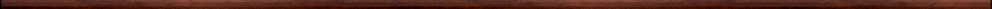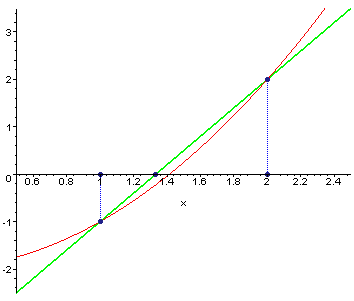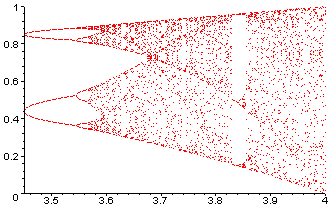Maple worksheets on numerical root-findingNumerical methods topics:

They are all compatible with Classic Worksheet Maple 10.The bisection method - bisect.mws

• A introduction to root-finding using graphs in conjunction with Maple's fsolve procedure.
• A procedure for finding a zero of a function by the bisection method: bisect.

Newton's method - newton.mws

• Explanation of Newton's method.
• A procedure for graphing successive steps of Newton's method: newton_step
• A procedure for finding a zero of a function by Newton's method: newton.

The secant method - secant.mws

• Explanation of the secant method for root finding.
• A procedure for graphing successive steps of the secant method: secant_step
• A procedure for finding a zero of a function by the secant method: secant.

Halley's method - halley.mws

• The iterative formula for Halley's method.
• Osculating hyperbolas and the Halley iteration formula.
• A procedure for graphing successive steps of the secant method: halley_step
• A procedure implementing Halley's method for root-finding: halley

The improved "leap-frog" Newton method for root-finding - LFnewton.mws

• The iterative formula for the improved "leap-frog" Newton method.
• A procedure for graphing successive steps of the improved "leap-frog" Newton method: impnewton_step
• A procedure for finding a zero of a function by the improved "leap-frog" Newton method: impnewton.

Brent's method for root-finding - brent.mws

• Inverse quadratic interpolation and Brent's method.
• A procedure for finding a zero of a function by Brent's method: brent.

General fixed point iteration for root-finding - itersolve.mws

• Hero's formula for calculating square roots
• Iterative formulas for solving equations
• A procedure for finding a fixed point of a real function: fixedpoint.
• Graphical illustration of iterative solutions
• Oscillating values from an iterative formula

A procedure for finding all roots in an interval where a sign change occurs - allbisect.mws

• A procedure for finding all zeros of a function in an interval where a sign change occurs: allbisect.
A bisection method is first used to pin down each root to a small interval.

A procedure for finding all roots in an interval - allroots.mws

• A procedure for finding all the zeros of a function in an interval: allroots.
It is a modification of the procedure allsolve written by Robert Israel from U.B.C.
It makes use of range arithmetic via Maple's evalr.

Numerical determination of a local maximum or minimum - maxmin.mws

• Golden section search for a maximum or minimum.
• Pictorial example of golden section search.
• Procedures which search for a local maximum or minimum: goldmax/goldmin.
• Searching for a maximum or minimum using parabolic interpolation.
• Pictorial example using parabolic interpolation.
• Procedures which search for a local maximum or minimum: findmax/findmin.

The logistic family of functions and chaos - chaos.mws

• The logistic family of functions.
• Iterating members of the logistic family.
• Bifurcation
• A procedure for displaying the bifurcation diagram of the logistic family: logisticdisplay.
• The bifurcation diagram of the logistic family.
• Chaos and islands of regularity.
• Finding a parameter value for the 3-cycle.
• Finding a parameter value for the 1st 5-cycle.
• Finding parameter values for the other two 5-cycles.

Root-finding procedures - roots.zipTop of page

Main index